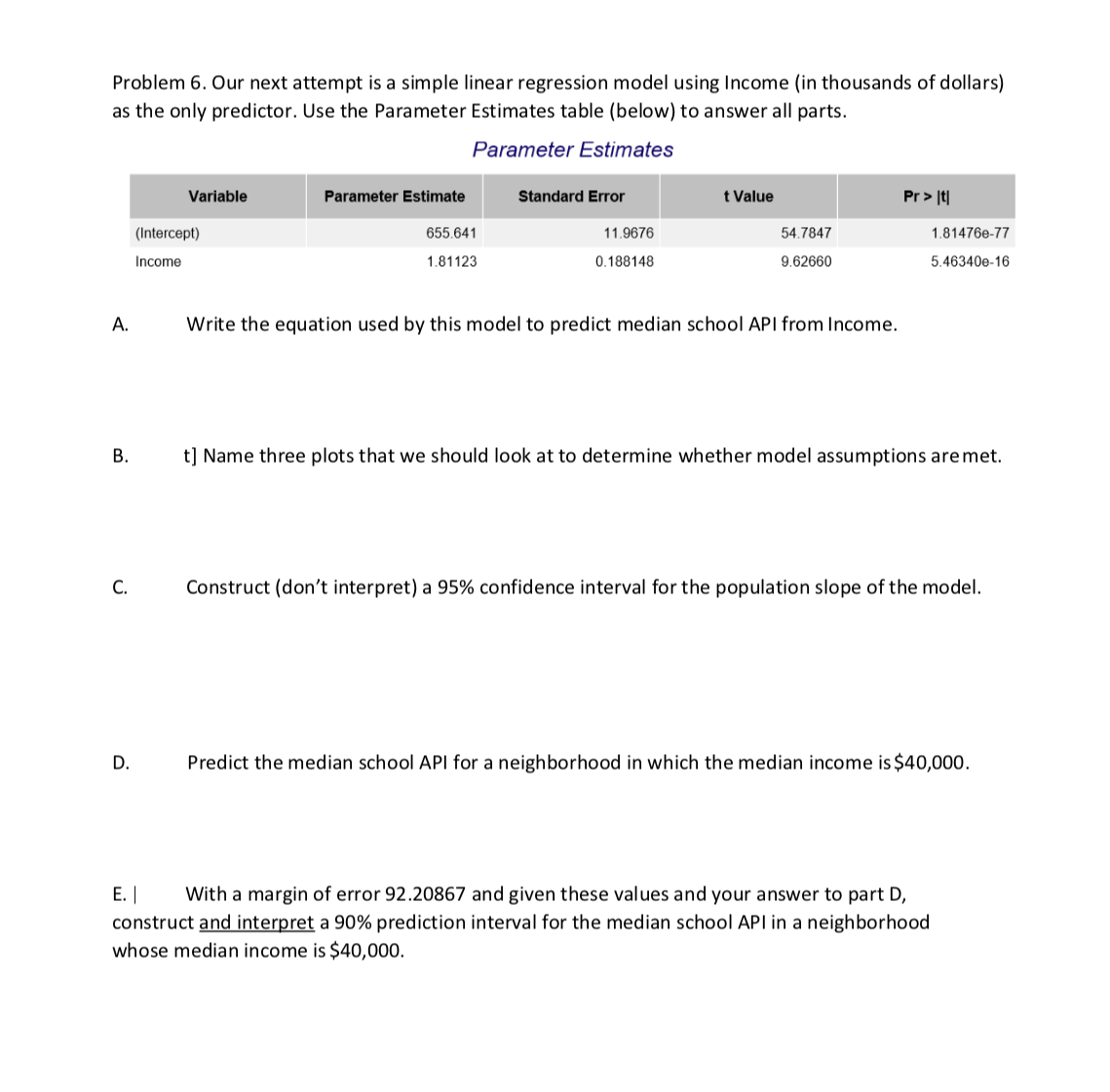# Problem 6. Our next attempt is a simple linear regression model using Income (in thousands of dollars)as the only predictor. Use the Parameter Estimates table (below) to answer all parts.Parameter EstimatesVariablet ValuePr >Parameter EstimateStandard Error655.64111.967654.7847(Intercept)1.81476e-771.811230.1881489.626605.46340e-16IncomeA.Write the equation used by this model to predict median school API from Income.t] Name three plots that we should look at to determine whether model assumptions are met.Construct (don't interpret) a 95% confidence interval for the population slope of the modelPredict the median school API for a neighborhood in which the median income is \$40,000.D.E.With a margin of error 92.20867 and given these values and your answer to part D,construct and interpret a 90% prediction interval for the median school API in a neighborhoodwhose median income is \$40,000

Question

questions d and ehelp_outlineImage TranscriptioncloseProblem 6. Our next attempt is a simple linear regression model using Income (in thousands of dollars) as the only predictor. Use the Parameter Estimates table (below) to answer all parts. Parameter Estimates Variable t Value Pr > Parameter Estimate Standard Error 655.641 11.9676 54.7847 (Intercept) 1.81476e-77 1.81123 0.188148 9.62660 5.46340e-16 Income A. Write the equation used by this model to predict median school API from Income. t] Name three plots that we should look at to determine whether model assumptions are met. Construct (don't interpret) a 95% confidence interval for the population slope of the model Predict the median school API for a neighborhood in which the median income is \$40,000. D. E.With a margin of error 92.20867 and given these values and your answer to part D, construct and interpret a 90% prediction interval for the median school API in a neighborhood whose median income is \$40,000 fullscreen
check_circle

Step 1

Part D:

The aim is to predict the median school API for a neighbourhood in which the median income is \$40,000.

Step 2

Denote y as the response variable, median school API and x as the predictor, income. In the given output, the column “Parameter Estimate” gives the coefficient corresponding to the variable under the “Variable” column.

The ‘estimate’ of “Intercept” is the intercept of the regression model, which is 655.641. The coefficient of “Income” is the slope, which is given as 1.81123. Using these values, the equation for predicting y using x is: y = 655.641 + 1.81123 x.

Here, x = 40 (in thousand dollars)

Step 3

Part E:

The aim is to find the 90% prediction interval for the median school API for a neighbourhood in whose median income is \$40,000.

We assume that the margin of error, E of 92.20867 ...

### Want to see the full answer?

See Solution

#### Want to see this answer and more?

Solutions are written by subject experts who are available 24/7. Questions are typically answered within 1 hour.*

See Solution
*Response times may vary by subject and question.
Tagged in

### Statistics